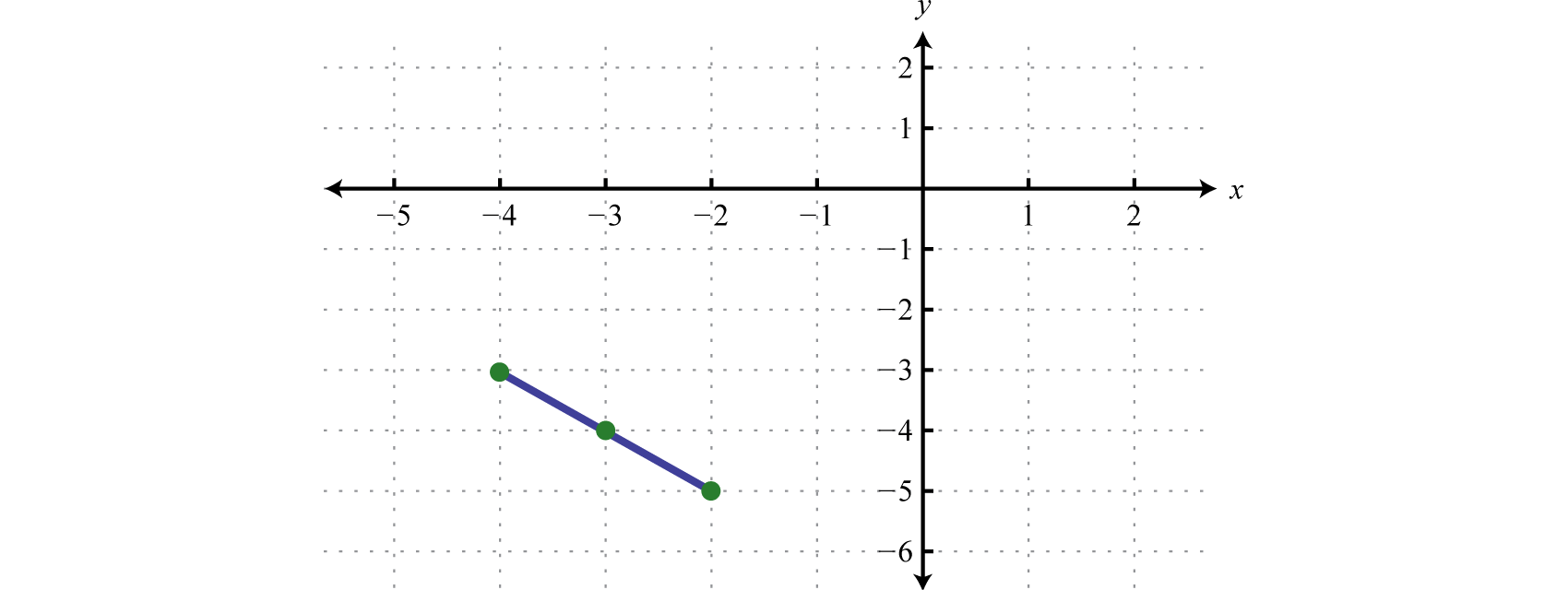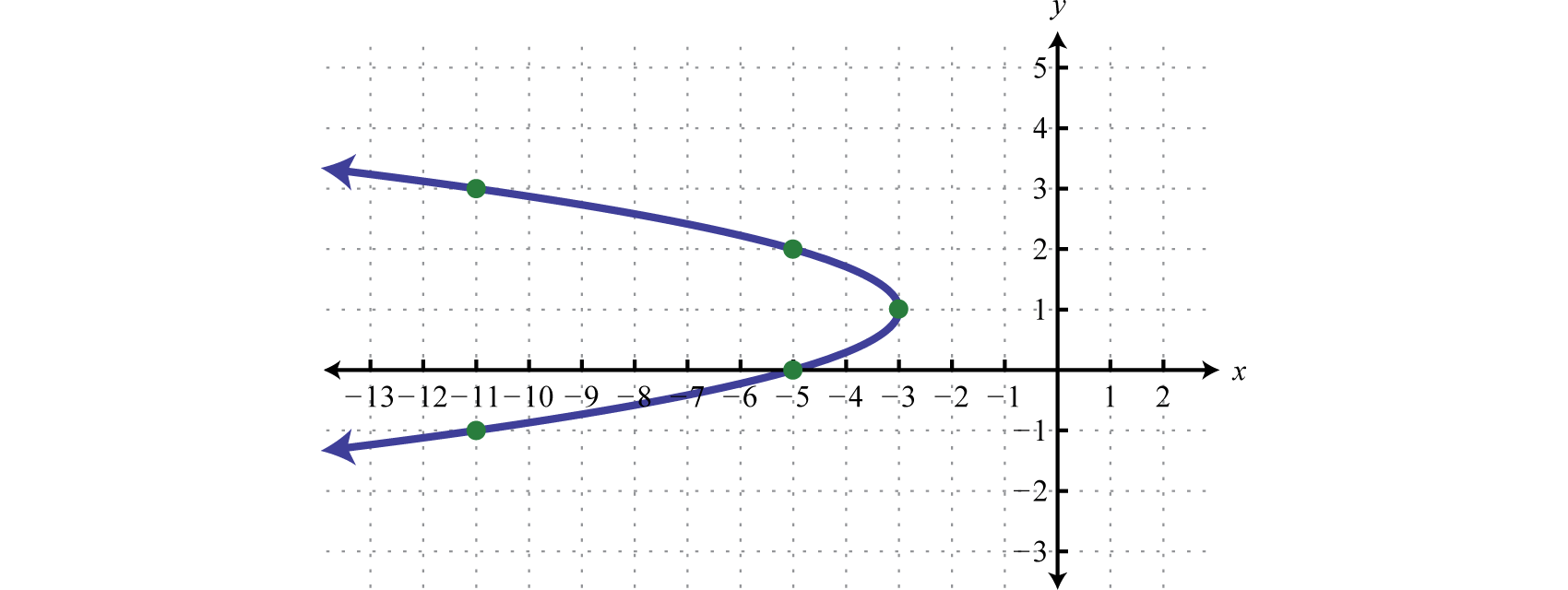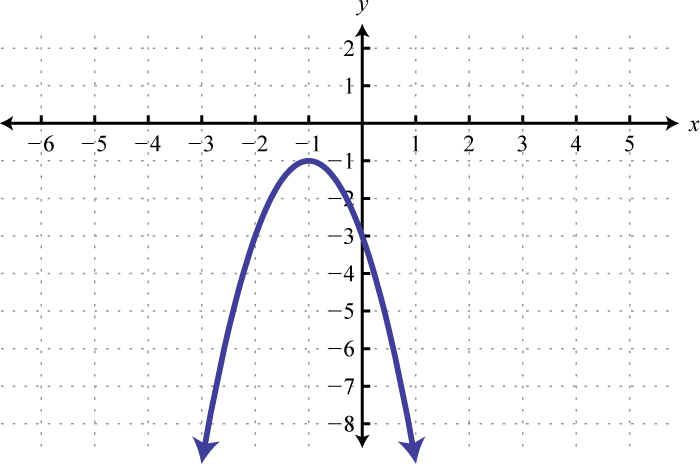$$\newcommand{\id}{\mathrm{id}}$$ $$\newcommand{\Span}{\mathrm{span}}$$ $$\newcommand{\kernel}{\mathrm{null}\,}$$ $$\newcommand{\range}{\mathrm{range}\,}$$ $$\newcommand{\RealPart}{\mathrm{Re}}$$ $$\newcommand{\ImaginaryPart}{\mathrm{Im}}$$ $$\newcommand{\Argument}{\mathrm{Arg}}$$ $$\newcommand{\norm}{\| #1 \|}$$ $$\newcommand{\inner}{\langle #1, #2 \rangle}$$ $$\newcommand{\Span}{\mathrm{span}}$$

8.1: Distance, Midpoint, and the Parabola

$$\newcommand{\vecs}{\overset { \rightharpoonup} {\mathbf{#1}} }$$

$$\newcommand{\vecd}{\overset{-\!-\!\rightharpoonup}{\vphantom{a}\smash {#1}}}$$

Skills to Develop

• Apply the distance and midpoint formulas.
• Graph a parabola using its equation given in standard from.
• Determine standard form for the equation of a parabola given general form.

Conic Sections

A conic section1 is a curve obtained from the intersection of a right circular cone and a plane. The conic sections are the parabola, circle, ellipse, and hyperbola.Figure 8.1.1

The goal is to sketch these graphs on a rectangular coordinate plane.Figure 8.1.2

The Distance and Midpoint Formulas

We begin with a review of the distance formula2. Given two points $$(x_{1}, y_{1})$$  and $$( x_{2}, y_{2})$$  in a rectangular coordinate plane, the distance $$d$$ between them is given by the distance formula,

$$d=\sqrt{\left(x_{2}-x_{1}\right)^{2}+\left(y_{2}-y_{1}\right)^{2}}$$

Furthermore, the point that bisects the line segment formed by these two points is called the midpoint3 and is given by the formula,

$$\left(\frac{x_{1}+x_{2}}{2}, \frac{y_{1}+y_{2}}{2}\right)$$

The midpoint is an ordered pair formed by the average of the $$x$$-values and the average of the $$y$$-values.

Example $$\PageIndex{1}$$:

Given $$(−2,−5)$$ and $$(−4,−3)$$ calculate the distance and midpoint between them.

Solution:

In this case, we will use the formulas with the following points:

$$\begin{array}{c c}{\left(x_{1}, y_{1}\right)} &{\left(x_{2}, y_{2}\right)} \\ {\color{black}{(\color{Cerulean}{-2}\color{black}{,}\color{OliveGreen}{-5})}}&{\color{black}{(\color{Cerulean}{-4}\color{black}{,}\color{OliveGreen}{-3})}}\end{array}$$

It is a good practice to include the formula in its general form before substituting values for the variables; this improves readability and reduces the probability of making errors.

\begin{aligned} d &=\sqrt{\left(x_{2}-x_{1}\right)^{2}+\left(y_{2}-y_{1}\right)^{2}} \\ &=\sqrt{[\color{Cerulean}{-4}\color{black}{-}(\color{Cerulean}{-2}\color{black}{)}]^{2}+[\color{OliveGreen}{-3}\color{black}{-}(\color{OliveGreen}{-5}\color{black}{)}]^{2}} \\ &=\sqrt{(-4+2)^{2}+(-3+5)^{2}} \\ &=\sqrt{(-2)^{2}+(2)^{2}} \\ &=\sqrt{4+4} \\ &=\sqrt{8} \\ &=2 \sqrt{2} \end{aligned}

Next determine the midpoint.

\begin{aligned}\left(\frac{x_{1}+x_{2}}{2}, \frac{y_{1}+y_{2}}{2}\right) &=\left(\frac{-2+(-4)}{2}, \frac{-5+(-3)}{2}\right) \\ &=\left(\frac{-6}{2}, \frac{-8}{2}\right) \\ &=(-3,-4) \end{aligned}

Plotting these points on a graph we have,Figure 8.1.3

Distance: $$2\sqrt{2}$$  units; midpoint: $$(−3,−4)$$

Example $$\PageIndex{2}$$:

The diameter of a circle is defined by the two points $$(−1,2)$$ and $$(1,−2)$$. Determine the radius of the circle and use it to calculate its area.

Solution:

Find the diameter using the distance formula.

\begin{aligned} d &=\sqrt{\left(x_{2}-x_{1}\right)^{2}+\left(y_{2}-y_{1}\right)^{2}} \\ &=\sqrt{\left[\color{Cerulean}{1}\color{black}{-}(\color{Cerulean}{-1}\color{black}{)}^{2}+(\color{OliveGreen}{-2}\color{black}{-}\color{OliveGreen}{2}\color{black}{)}^{2}\right.} \\ &=\sqrt{[2)^{2}+(-4)^{2}} \\&=\sqrt{4+16} \\&=\sqrt{20} \\ &=2 \sqrt{5} \end{aligned}

Recall that the radius of a circle is one-half of the circle’s diameter. Therefore, if $$d=2\sqrt{5}$$ units, then

$$r=\frac{d}{2}=\frac{2 \sqrt{5}}{2}=\sqrt{5}$$

The area of a circle is given by the formula $$A=πr^{2}$$ and we have

\begin{aligned} A &=\pi(\sqrt{5})^{2} \\ &=\pi \cdot 5 \\ &=5 \pi \end{aligned}

Area is measured in square units.

Radius: $$\sqrt{5}$$ units; area: $$5π$$ square units

Exercise $$\PageIndex{1}$$

Given $$(0,0)$$ and $$(9,−3)$$ calculate the distance and midpoint between them.

Distance: $$3\sqrt{10}$$ units; midpoint: $$\left(\frac{9}{2},-\frac{3}{2}\right)$$

The Parabola

A parabola4 is the set of points in a plane equidistant from a given line, called the directrix, and a point not on the line, called the focus. In other words, if given a line $$L$$ the directrix, and a point $$F$$ the focus, then $$(x,y)$$ is a point on the parabola if the shortest distance from it to the focus and from it to the line is equal as pictured below:Figure 8.1.4

The vertex of the parabola is the point where the shortest distance to the directrix is at a minimum. In addition, a parabola is formed by the intersection of a cone with an oblique plane that is parallel to the side of the cone:Figure 8.1.5

Recall that the graph of a quadratic function, a polynomial function of degree 2, is parabolic. We can write the equation of a parabola in general form5 or we can write the equation of a parabola in standard form6:

$$\begin{array}{cc}{\color{Cerulean} { General\: Form }} & {\color{Cerulean} { Standard\: Form }} \\ {y=a x^{2}+b x+c} & {y=a(x-h)^{2}+k}\end{array}$$

Both forms are useful in determining the general shape of the graph. However, in this section we will focus on obtaining standard form, which is often called vertex form7. Given a quadratic function in standard form, the vertex is $$(h,k)$$. To see that this is the case, consider graphing $$y=(x+3)^{2}+2$$ using transformations.

$$\begin{array}{ll}{y=x^{2}} & {\color{Cerulean} { Basic\: squaring\: function. }} \\ {y=(x+3)^{2}} & {\color{Cerulean} {Horizontal\: shift\: left\: 3\: units. }} \\ {y=(x+3)^{2}+2} & {\color{Cerulean} { Vertical\: shift\: up\: 2\: units. }}\end{array}$$

Use these translations to sketch the graph,Figure 8.1.6

Here we can see that the vertex is $$(−3,2)$$. This can be determined directly from the equation in standard form,

$$\begin{array}{l}{y=a(x-h)^{2}\:+\:\:k} \\\color{Cerulean}{\quad\quad\quad\quad\:\downarrow \quad\quad\: \color{Cerulean}{\downarrow}} \\ {y=[x-(-3)]^{2}+2}\end{array}$$

Written in this form we can see that the vertex is $$(−3,2)$$. However, the equation is typically not given in standard form. Transforming general form to standard form, by completing the square, is the main process by which we will sketch all of the conic sections.

Example $$\PageIndex{3}$$:

Rewrite the equation in standard form and determine the vertex of its graph: $$y=x^{2}-8 x+15$$.

Solution:

Begin by making room for the constant term that completes the square.

\begin{aligned} y &=x^{2}-8 x+15 \\ &=x^{2}-8 x \color{Cerulean}{+ \_\_\_ }\color{black}{+}15 \color{Cerulean}{- \_\_\_}\end{aligned}

The idea is to add and subtract the value that completes the square, $$\left(\frac{b}{2}\right)^{2}$$, and then factor. In this case, add and subtract $$\left(\frac{b}{2}\right)^{2}=\left(\frac{-8}{2}\right)^{2}=(-4)^{2}=16$$.

\begin{aligned} y &=x^{2}-8 x+15 \quad\quad\quad\quad\quad\quad\:\:\color{Cerulean}{Add\:and\:subtract\:16.} \\ &=\color{black}{\left(x^{2}-8 x\color{Cerulean}{+16}\right)}+15\color{Cerulean}{-16}\quad\color{Cerulean}{Factor.} \\ &=(x-4)(x-4)-1 \\ &=(x-4)^{2}-1 \end{aligned}

Adding and subtracting the same value within an expression does not change it. Doing so is equivalent to adding $$0$$. Once the equation is in this form, we can easily determine the vertex.

$$\begin{array}{l}{y=a(x-h)^{2}\:+\:k} \\\quad\quad\quad\quad\:\color{Cerulean}{\downarrow}\quad\quad\color{Cerulean}{\downarrow} \\ {y=(x\:-\:4)^{2}+(-1)}\end{array}$$

Here we have a translation to the right $$4$$ units and down $$1$$ unit. Hence, $$h = 4$$ and $$k = −1$$.

$$y=(x-4)^{2}-1$$; vertex: $$(4,−1)$$

If there is a leading coefficient other than $$1$$, then begin by factoring out that leading coefficient from the first two terms of the trinomial.

Example $$\PageIndex{4}$$:

Rewrite the equation in standard form and determine the vertex of the graph: $$y=-2 x^{2}+12 x-16$$.

Solution:

Since $$a=−2$$, factor this out of the first two terms in order to complete the square. Leave room inside the parentheses to add and subtract the value that completes the square.

\begin{aligned} y &=-2 x^{2}+12 x-16 \\ &=-2\color{black}{\left(x^{2}-6 x\color{Cerulean}{+\_\_\_-\_\_\_}\right)-}16\end{aligned}

Now use $$−6$$ to determine the value that completes the square. In this case, $$\left(\frac{b}{2}\right)^{2}=\left(\frac{-6}{2}\right)^{2}=(-3)^{2}=9$$. Add and subtract $$9$$ and factor as follows:

\begin{aligned} y &=-2 x^{2}+12 x-16 \\ &=-2\color{black}{\left(x^{2}-6 x\color{Cerulean}{+\_\_\_-\_\_\_}\right)}-16\quad\color{Cerulean}{Add\:and\:subtract\:9.} \\ &=-2\color{black}{\left(x^{2}-6 x\color{Cerulean}{+9-9}\right)-}16\quad\quad\:\:\quad\color{Cerulean}{Factor.} \\ &=-2[(x-3)(x-3)-9]-16\ \\ &=-2\left[(x-3)^{2}-9\right]-16\quad\quad\quad\quad\:\:\color{Cerulean}{Distribute\:the\:-2.} \\ &=-2(x-3)^{2}+18-16 \\ &=-2(x-3)^{2}+2 \end{aligned}

In this form, we can easily determine the vertex.

$$\begin{array}{l}{y=a\:(x\:-\:h)^{2}+k}\\\quad\quad\quad\quad\quad\color{Cerulean}{\downarrow}\quad\:\:\color{Cerulean}{\downarrow} \\ {y=-2(x-3)^{2}+2}\end{array}$$

Here $$h = 3$$ and $$k = 2$$.

y=−2(x−3)2+2y=−2(x−3)2+2; vertex: (3,2)(3,2)

Make use of both general form and standard form when sketching the graph of a parabola.

Example $$\PageIndex{5}$$:

Graph: $$y=-2 x^{2}+12 x-16$$.

Solution:

From the previous example we have two equivalent forms of this equation,

$$\begin{array}{c} {\color{Cerulean}{General\:Form}}&{\color{Cerulean}{Standard\:Form}}\\{y=-2 x^{2}+12 x-16}&{ y=-2(x-3)^{2}+2}\end{array}$$

Recall that if the leading coefficient $$a>0$$ the parabola opens upward and if $$a<0$$ the parabola opens downward. In this case, $$a=−2$$ and we conclude the parabola opens downward. Use general form to determine the $$y$$-intercept. When $$x=0$$ we can see that the $$y$$-intercept is $$(0,−16)$$. From the equation in standard form, we can see that the vertex is $$(3,2)$$. To find the $$x$$-intercept we could use either form. In this case, we will use standard form to determine the $$x$$-values where $$y=0$$,

\begin{aligned} y &=-2(x-3)^{2}+2\quad\color{Cerulean}{Set\:y=0\:and\:solve.} \\ 0 &=-2(x-3)^{2}+2 \\-2 &=-2(x-3)^{2} \\ & 1=(x-3)^{2}\quad\quad\quad\:\:\color{Cerulean}{Apply\:the\:square\:root\:property.} \\ \pm 1 &=x-3 \\ 3 \pm 1 &=x \end{aligned}

Here $$x=3−1=2$$ or $$x=3+1=4$$ and therefore the $$x$$-intercepts are $$(2,0)$$ and $$(4,0)$$. Use this information to sketch the graph.Figure 8.1.7

So far we have been sketching parabolas that open upward or downward because these graphs represent functions. At this point we extend our study to include parabolas that open right or left. If we take the equation that defines the parabola in the previous example,

$$y=-2(x-3)^{2}+2$$

and switch the x and y values we obtain

$$x=-2(y-3)^{2}+2$$

This produces a new graph with symmetry about the line $$y=x$$.Figure 8.1.8

Note that the resulting graph is not a function. However, it does have the same general parabolic shape that opens left. We can recognize equations of parabolas that open left or right by noticing that they are quadratic in $$y$$ instead of $$x$$. Graphing parabolas that open left or right is similar to graphing parabolas that open upward and downward. In general, we haveFigure 8.1.9

In all cases, the vertex is $$(h,k)$$. Take care to note the placement of $$h$$ and $$k$$ in each equation.

Example $$\PageIndex{6}$$:

Graph: $$x=y^{2}+10 y+13$$.

Solution:

Because the coefficient of $$y^{2}$$ is positive, $$a=1$$, we conclude that the graph is a parabola that opens to the right. Furthermore, when $$y=0$$ it is clear that $$x=13$$ and therefore the $$x$$-intercept is $$(13,0)$$. Complete the square to obtain standard form. Here we will add and subtract $$\left(\frac{b}{2}\right)^{2}=\left(\frac{10}{2}\right)^{2}=(5)^{2}=25$$.

\begin{aligned} x &=y^{2}+10 y+13 \\ &=y^{2}+10 y\color{Cerulean}{+25-25}\color{black}{+}13 \\ &=(y+5)(y+5)-12 \\ &=(y+5)^{2}-12 \end{aligned}

Therefore,

$$\begin{array}{l}{x=a\:(y\:-\:k)^{2}\:\:\:+\:\:\:\:h}\\ \quad\quad\quad\quad\quad\color{Cerulean}{\downarrow}\quad\quad\quad\color{Cerulean}{\downarrow} \\ {x=(y-(-5))^{2}+(-12)}\end{array}$$

From this we can see that the vertex $$(h,k)=(−12,−5)$$. Next use standard form to find the $$y$$-intercepts by setting $$x=0$$.

\begin{aligned} x &=(y+5)^{2}-12 \\ 0 &=(y+5)^{2}-12 \\ 12 &=(y+5)^{2} \\ \pm \sqrt{12} &=y+5 \\ \pm 2 \sqrt{3} &=y+5 \\-5 \pm 2 \sqrt{3} &=y \end{aligned}

The $$y$$-intercepts are $$(0,-5-2 \sqrt{3})$$ and $$(0,-5+2 \sqrt{3})$$. Use this information to sketch the graph.Figure 8.1.10

Example $$\PageIndex{7}$$

Graph: $$x=-2 y^{2}+4 y-5$$.

Solution:

Because the coefficient of $$y^{2}$$ is $$a=−2$$, we conclude that the graph is a parabola that opens to the left. Furthermore, when $$y=0$$ it is clear that $$x=−5$$ and therefore the $$x$$-intercept is $$(−5,0)$$. Begin by factoring out the leading coefficient as follows:

\begin{aligned} x &=-2 y^{2}+4 y-5 \\ &=-2\left(y^{2}-2 y+\_\_\_-\_\_\_\right)-5\end{aligned}

Here we will add and subtract $$\left(\frac{b}{2}\right)^{2}=\left(\frac{-2}{2}\right)^{2}=(-1)^{2}=1$$.

\begin{aligned} x &=-2 y^{2}+4 y-5 \\ &=-2\color{black}{\left(y^{2}-2 y\color{Cerulean}{+1-1}\right)-}5 \\ &=-2\left[(y-1)^{2}-1\right]-5 \\ &=-2(y-1)^{2}+2-5 \\ &=-2(y-1)^{2}-3 \end{aligned}

Therefore, from vertex form, $$x=-2(y-1)^{2}-3$$, we can see that the vertex is $$(h,k)=(−3,1)$$. Because the vertex is at $$(−3,1)$$ and the parabola opens to the left, we can conclude that there are no $$y$$-intercepts. Since we only have two points, choose some $$y$$-values and find the corresponding $$x$$-values.

 $$x$$ $$y$$ $$x=-2(y-1)^{2}-3$$ $$\color{Cerulean}{-11}$$ $$-1$$ $$x=-2(-1-1)^{2}-3=-2(-2)^{2}-3=-11$$ $$\color{Cerulean}{-5}$$ $$2$$ $$x=-2(2-1)^{2}-3=-2(1)^{2}-3=-5$$ $$\color{Cerulean}{-11}$$ $$3$$ $$x=-2(3-1)^{2}-3=-2(2)^{2}-3=-11$$

Table 8.1.1Figure 8.1.11

Exercise $$\PageIndex{2}$$:

Graph: $$x= y^{2}−y−6$$.Figure 8.1.12

Key Takeaways

• Use the distance formula to determine the distance between any two given points. Use the midpoint formula to determine the midpoint between any two given points.
• A parabola can open upward or downward, in which case, it is a function. In this section, we extend our study of parabolas to include those that open left or right. Such graphs do not represent functions.
• The equation of a parabola that opens upward or downward is quadratic in $$x, y=ax^{2}+bx+c$$. If $$a>0$$, then the parabola opens upward and if a<0a<0, then the parabola opens downward.
• The equation of a parabola that opens left or right is quadratic in $$y, x=ay^{2}+by+c$$. If $$a>0$$, then the parabola opens to the right and if $$a<0$$, then the parabola opens to the left.
• The equation of a parabola in general form $$y=ax^{2}+bx+c$$ or $$x=ay^{2}+by+c$$ can be transformed to standard form $$y=a(x−h)^{2}+k$$ or $$x=a(y−k)^{2}+h$$ by completing the square.
• When completing the square, ensure that the leading coefficient of the variable grouping is $$1$$ before adding and subtracting the value that completes the square.
• Both general and standard forms are useful when graphing parabolas. Given standard form the vertex is apparent $$(h,k)$$. To find the $$x$$-intercept set $$y=0$$ and solve for $$x$$ and to find the $$y$$-intercept set $$x=0$$ and solve for $$y$$.

Exercise $$\PageIndex{3}$$

Calculate the distance and midpoint between the given two points.

1. $$(-1,-3)$$ and $$(5,-11)$$
2. $$(-3,2)$$ and $$(1,-1)$$
3. $$(4,-2)$$ and $$(-2,-6)$$
4. $$(-5,-6)$$ and $$(-3,-4)$$
5. $$(10,-1)$$ and $$(9,6)$$
6. $$(-6,-4)$$ and $$(-12,1)$$
7. $$(0,0)$$ and $$(\sqrt{2}, \sqrt{3})$$
8. $$(0,0)$$ and $$(2 \sqrt{2},-\sqrt{3})$$
9. $$(\sqrt{5},-\sqrt{3})$$ and $$(2 \sqrt{5},-\sqrt{3})$$
10. $$(3 \sqrt{10}, \sqrt{6})$$ and $$(\sqrt{10},-5 \sqrt{6})$$
11. $$\left(\frac{1}{2},-1\right)$$ and $$\left(-2, \frac{3}{2}\right)$$
12. $$\left(-\frac{4}{3}, 2\right)$$ and $$\left(-\frac{1}{3},-\frac{1}{2}\right)$$
13. $$\left(\frac{1}{5},-\frac{9}{5}\right)$$ and $$\left(\frac{3}{10},-\frac{5}{2}\right)$$
14. $$\left(-\frac{1}{2}, \frac{4}{3}\right)$$ and $$\left(-\frac{2}{3}, \frac{5}{6}\right)$$
15. $$(a, b)$$ and $$(0,0)$$
16. $$(0,0)$$ and $$(a \sqrt{2}, 2 \sqrt{a})$$

1. Distance: $$10$$ units; midpoint: $$(2, −7)$$

3. Distance: $$2\sqrt{13}$$ units; midpoint: $$(1, −4)$$

5. Distance: $$5\sqrt{2}$$ units; midpoint: $$(\frac{19}{2},\frac{5}{2})$$

7. Distance: $$\sqrt{5}$$ units; midpoint: $$\left(\frac{\sqrt{2}}{2}, \frac{\sqrt{3}}{2}\right)$$

9. Distance: $$\sqrt{5}$$ units; midpoint: $$\left(\frac{3 \sqrt{5}}{2},-\sqrt{3}\right)$$

11. Distance: $$\frac{5 \sqrt{2}}{2}$$ units; midpoint: $$(−\frac{3}{4}, \frac{1}{4})$$

13. Distance: $$\frac{\sqrt{2}}{2}$$ units; midpoint: $$\left(\frac{1}{4},-\frac{43}{20}\right)$$

15. Distance: $$\sqrt{a^{2}+b^{2}}$$ units; midpoint: $$\left(\frac{a}{2}, \frac{b}{2}\right)$$

Exercise $$\PageIndex{4}$$

Determine the area of a circle whose diameter is defined by the given two points.

1. $$(-8,12)$$ and $$(-6,8)$$
2. $$(9,5)$$ and $$(9,-1)$$
3. $$(7,-8)$$ and $$(5,-10)$$
4. $$(0,-5)$$ and $$(6,1)$$
5. $$(\sqrt{6}, 0)$$ and $$(0,2 \sqrt{3})$$
6. $$(0, \sqrt{7})$$ and $$(\sqrt{5}, 0)$$

1. $$5π$$ square units

3. $$2π$$ square units

5. $$\frac{9}{2} π$$ square units

Exercise $$\PageIndex{5}$$

Determine the perimeter of the triangle given the coordinates of the vertices.

1. $$(5,3),(2,-3),$$ and $$(8,-3)$$
2. $$(-3,2),(-4,-1),$$ and $$(-1,0)$$
3. $$(3,3),(5,3-2 \sqrt{3}),$$ and $$(7,3)$$
4. $$(0,0),(0,2 \sqrt{2}),$$ and $$(\sqrt{2}, 0)$$

1. $$6+6 \sqrt{5}$$ units

3. $$12$$ units

Exercise $$\PageIndex{6}$$

Find $$a$$ so that the distance $$d$$ between the points is equal to the given quantity.

1. $$(1,2)$$ and $$(4, a) ; d=5$$ units
2. $$(-3, a)$$ and $$(5,6) ; d=10$$ units
3. $$(3,1)$$ and $$(a, 0) ; d=\sqrt{2}$$ units
4. $$(a, 1)$$ and $$(5,3) ; d=\sqrt{13}$$ units

1. $$-2,6$$

3. $$2,4$$

Exercise $$\PageIndex{7}$$

Graph. Be sure to find the vertex and all intercepts.

1. $$y=x^{2}+3$$
2. $$y=\frac{1}{2}(x-4)^{2}$$
3. $$y=-2(x+1)^{2}-1$$
4. $$y=-(x-2)^{2}+1$$
5. $$y=-x^{2}+3$$
6. $$y=-(x+1)^{2}+5$$
7. $$x=y^{2}+1$$
8. $$x=y^{2}-4$$
9. $$x=(y+2)^{2}$$
10. $$x=(y-3)^{2}$$
11. $$x=-y^{2}+2$$
12. $$x=-(y+1)^{2}$$
13. $$x=\frac{1}{3}(y-3)^{2}-1$$
14. $$x=-\frac{1}{3}(y+3)^{2}-1$$

1.Figure 8.1.13

3.Figure 8.1.14

5.Figure 8.1.15

7.Figure 8.1.16

9.Figure 8.1.17

11.Figure 8.1.18

13.Figure 8.1.19

Exercise $$\PageIndex{8}$$

Rewrite in standard form and give the vertex.

1. $$y=x^{2}-6 x+18$$
2. $$y=x^{2}+8 x+36$$
3. $$x=y^{2}+20 y+87$$
4. $$x=y^{2}-10 y+21$$
5. $$y=x^{2}-14 x+49$$
6. $$x=y^{2}+16 y+64$$
7. $$x=2 y^{2}-4 y+5$$
8. $$y=3 x^{2}-30 x+67$$
9. $$y=6 x^{2}+36 x+54$$
10. $$x=3 y^{2}+6 y-1$$
11. $$y=2 x^{2}-2 x-1$$
12. $$x=5 y^{2}+15 y+9$$
13. $$x=-y^{2}+5 y-5$$
14. $$y=-x^{2}+9 x-20$$

1. $$y=(x-3)^{2}+9 ;$$ vertex: $$(3,9)$$

3. $$x=(y+10)^{2}-13 ;$$ vertex: $$(-13,-10)$$

5. $$y=(x-7)^{2} ;$$ vertex: $$(7,0)$$

7. $$x=2(y-1)^{2}+3 ;$$ vertex: $$(3,1)$$

9. $$y=6(x+3)^{2} ;$$ vertex: $$(-3,0)$$

11. $$y=2\left(x-\frac{1}{2}\right)^{2}-\frac{3}{2} ;$$ vertex: $$\left(\frac{1}{2},-\frac{3}{2}\right)$$

13. $$x=-\left(y-\frac{5}{2}\right)^{2}+\frac{5}{4} ;$$ vertex: $$\left(\frac{5}{4}, \frac{5}{2}\right)$$

Exercise $$\PageIndex{9}$$

Rewrite in standard form and graph. Be sure to find the vertex and all intercepts.

1. $$y=x^{2}-4 x-5$$
2. $$y=x^{2}+6 x-16$$
3. $$y=-x^{2}+12 x-32$$
4. $$y=-x^{2}-10 x$$
5. $$y=2 x^{2}+4 x+9$$
6. $$y=3 x^{2}-6 x+4$$
7. $$y=-5 x^{2}+30 x-45$$
8. $$y=-4 x^{2}-16 x-16$$
9. $$x=y^{2}-2 y-8$$
10. $$x=y^{2}+4 y+8$$
11. $$x=y^{2}-2 y-3$$
12. $$x=y^{2}+6 y-7$$
13. $$x=-y^{2}-10 y-24$$
14. $$x=-y^{2}-12 y-40$$
15. $$x=3 y^{2}+12 y+12$$
16. $$x=-2 y^{2}+12 y-18$$
17. $$x=y^{2}-4 y-3$$
18. $$x=y^{2}+6 y+1$$
19. $$x=-y^{2}+2 y+5$$
20. $$y=2 x^{2}-2 x+1$$
21. $$y=-3 x^{2}+2 x+1$$
22. $$y=-x^{2}+3 x+10$$
23. $$x=-4 y^{2}-4 y-5$$
24. $$x=y^{2}-y+2$$
25. $$y=x^{2}+5 x-1$$
26. $$y=2 x^{2}+6 x+3$$
27. $$x=2 y^{2}+10 x+12$$
28. $$x=y^{2}+y-1$$

1. $$y=(x-2)^{2}-9$$;Figure 8.1.20

3. $$y=-(x-6)^{2}+4$$;Figure 8.1.21

5. $$y=2(x+1)^{2}+7$$;Figure 8.1.22

7. $$y=-5(x-3)^{2}$$;Figure 8.1.23

9. $$x=(y-1)^{2}-9$$;Figure 8.1.24

11. $$x=(y-1)^{2}-4$$;Figure 8.1.25

13. $$x=-(y+5)^{2}+1$$;Figure 8.1.26

15. $$x=3(y+2)^{2}$$;Figure 8.1.27

17. $$x=(y-2)^{2}-7$$;Figure 8.1.28

19. $$x=-(y-1)^{2}+6$$;Figure 8.1.29

21. $$y=-3\left(x-\frac{1}{3}\right)^{2}+\frac{4}{3}$$;Figure 8.1.30

23. $$x=-4\left(y+\frac{1}{2}\right)^{2}-4$$;Figure 8.1.31

25. $$y=\left(x+\frac{5}{2}\right)^{2}-\frac{29}{4}$$;Figure 8.1.32

27. $$x=2\left(y+\frac{5}{2}\right)^{2}-\frac{1}{2}$$;Figure 8.1.33

Exercise $$\PageIndex{10}$$

1. Research and discuss real-world applications that involve a parabola.
2. Do all parabolas have $$x$$-intercepts? Explain.
3. Do all parabolas have $$y$$-intercepts? Explain.
4. Make up your own parabola that opens left or right, write it in general form, and graph it.

Footnotes

1A curve obtained from the intersection of a right circular cone and a plane.

2Given two points $$(x_{1} , y_{1})$$ and $$(x_{2}, y_{2})$$, the distance $$d$$ between them is given by $$d=\sqrt{\left(x_{2}-x_{1}\right)^{2}+\left(y_{2}-y_{1}\right)^{2}}$$.

3Given two points $$(x_{1}, y_{1})$$ and $$(x_{2}, y_{2})$$, the midpoint is an ordered pair given by $$\left(\frac{x_{1}+x_{2}}{2}, \frac{y_{1}+y_{2}}{2}\right)$$.

4The set of points in a plane equidistant from a given line, called the directrix, and a point not on the line, called the focus.

5The equation of a parabola written in the form $$y=a x^{2}+b x+c$$ or $$x=a y^{2}+b y+c$$, where $$a, b$$, and $$c$$ are real numbers and $$a ≠ 0$$.

6The equation of a parabola written in the form $$y=a(x-h)^{2}+k$$ or $$x=a(y-k)^{2}+h$$.

7The equation of a parabola written in standard form is often called vertex form. In this form the vertex is apparent: $$(h, k)$$.